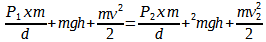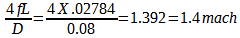# Speed of air flow in pipe

SpiraRoam

## Homework Statement

A tank with a 25.5 litre capacity contains air compressed into 355 atmospheres used to drive a torpedo by expansion into an engine via a pipe of diameter 0.08cm. A mechanism ensures that the gas flows to the engine at a constant rate and any cooling of the air during expansion to the 1 atmosphere pressure in the pipe can be neglected.

If the engine is driven by the air for 12.0 minutes until the tank is empty, at what speed does the air flow in the pipe?

## Homework EquationsBernoulli's Equation

P1/p = P2/p + V"/2 which produces V"2 = square root of ((P1 - P2)/p x 2## The Attempt at a Solution

I'm not sure whether it's a mixture of thermal dynamics and thermal effects in gases. I don't know whether to bring in Pascals law or the pressure / temperature / density relationship and gas properties / heat capacities? I did a lot of reading into Intro to fluid dynamics by Stanley Middleman - that's where I also worked out a mach number of 1.4 from for the air.

My scenario is abit different than the ones I've read on here / in the books. The Stanley Middleman book at pg 479 doesn't actually give an answer to the air speed - it starts talking about entropy and all sorts of other things I'm not sure are relevant?

I just want to know the airflow speed for 25.5litres of compressed air to 355 atmospheres which takes 12 minutes to empty through a 0.08cm diameter pipe?! Why is it so hard to relate it all?Thanks

#### Attachments

Mentor
What is the number of moles of air initially inside the tank, taking into account non-ideal gas effects? What volume does this number of moles of air occupy at 1 atm and room temperature?

SpiraRoam
It doesn't say. All I've been given is what's in the problem statement. I'm off to work now - I'll reply tomorrow, cheers

Mentor
It doesn't say. All I've been given is what's in the problem statement. I'm off to work now - I'll reply tomorrow, cheers
Are you saying that, if you know the pressure, volume, and temperature, you can't calculate the number of moles?

SpiraRoam
I honestly don't know much about moles, I'll have to research. There's no indication of a temperature though - it just says that a cooling takes place for it to get to standard atmosphere pressure and that it's negligible. Is it possible to find moles with just the pressure and volume?

Mentor
I honestly don't know much about moles, I'll have to research. There's no indication of a temperature though - it just says that a cooling takes place for it to get to standard atmosphere pressure and that it's negligible. Is it possible to find moles with just the pressure and volume?
You need to assume room temperature.

SpiraRoam
Okay, I didn't know you could make assumptions outside of the stated variables in solving problems - I thought you had to stick to exactly what was told in Engineering scenarios. It's based on the inside of a tank- would that qualify as 'room' temperature?

To find the initial number of moles for the air at 355 I need to first calculate the temperature of the air at that atm and then use the ideal gas equation? I repeat this for the standard room temperature. I need to do some reading on moles before I do that. How do I apply the difference in moles to the initial problem of airflow speed?

Thanks

Mentor
Sorry. It may not be necessary to determine the number of moles if it is treated as an ideal gas. If you expand 25.5 liters of gas from 355 atm to 1 atm at constant temperature, from the ideal gas law, what is the new volume? If it takes 12 minutes for the gas to flow this volume of gas at constant rate through the pipe, what is the volumetric flow rate per second?

SpiraRoam
In order to find the volumes of each atm using the ideal gas law I need to have the amount of moles (n), R - the ideal gas constant for the 355 and T the temperature in Kelvins. I'm not sure how to find them, do you have any quick solutions - to save me a load of reading? XD

It's just for the initial pressure of 355 atm, finding those values for 1 atm should be fine. I assume the 'new volume' after expansion is the difference in volume between the 355atm and 1atm pressures?

Thanks

Mentor
In order to find the volumes of each atm using the ideal gas law I need to have the amount of moles (n), R - the ideal gas constant for the 355 and T the temperature in Kelvins. I'm not sure how to find them, do you have any quick solutions - to save me a load of reading? XD

It's just for the initial pressure of 355 atm, finding those values for 1 atm should be fine. I assume the 'new volume' after expansion is the difference in volume between the 355atm and 1atm pressures?

Thanks
(26.5)(355)=V liters

SpiraRoam
So I'm not actually using the ideal gas equation to find the original Volume in liters, just multiplying the atmosphere by the 25.5l? How come it's okay to do that? By that working out the volume for 1atm would just be (25.5)(1)=V liters

Mentor
So I'm not actually using the ideal gas equation to find the original Volume in liters, just multiplying the atmosphere by the 25.5l? How come it's okay to do that? By that working out the volume for 1atm would just be (25.5)(1)=V liters
If the temperature and the number of moles is constant, then the ideal gas law reduces to Boyle's Law, ##P_2V_2=P_1V_1##, where ##P_1=355\ atm##, ##V_1= 25.5\ liters##, ##P_2=1\ atm##, and ##V_2=??\ liters##.

SpiraRoam
I went to see my tutor earlier and that's what he said. He said V2 should be around 6m^3

In finding V2 from the equation it's P1V1/P2 right? Would the value of P2 need to change from 1 atm to the equivalent atm / L? Otherwise we've got 9052.50 atmosphere liters / 1 atm? I'm missing something here and Khan Academy isn't giving me the right info on how to apply Boyles law.

I've got 7 more questions for this coursework to be in by the 12th, I need to get a move on haha

Mentor
I went to see my tutor earlier and that's what he said. He said V2 should be around 6m^3

In finding V2 from the equation it's P1V1/P2 right? Would the value of P2 need to change from 1 atm to the equivalent atm / L? Otherwise we've got 9052.50 atmosphere liters / 1 atm?
You are aware that the units of atm in the numerator and the denominator cancel out, right? You're also aware that 9000 liters is 9 m^3, right?

Last edited:
SpiraRoam
No I wasn't, cheers for saying! So I've got 9052.50 which l put into the Vol flow rate equation for a volume flow rate of 12.573 m^3/s.

I then found the cross sectional area of the pipe by A=pi r^2 which is:

0.08cm/2 = 0.04cm = pi x 0.04^2 = 0.005026548 m^2

which I then plugged into the speed of flow equation: flow rate / cross sectional area for

12.573m^3/s divided by .005026548m^2 = 2501.319m^3/s

would you say 3 significant figures is better than 2? Or is that unnecessary?

Mentor
No I wasn't, cheers for saying! So I've got 9052.50 which l put into the Vol flow rate equation for a volume flow rate of 12.573 m^3/s.

I then found the cross sectional area of the pipe by A=pi r^2 which is:

0.08cm/2 = 0.04cm = pi x 0.04^2 = 0.005026548 m^2

which I then plugged into the speed of flow equation: flow rate / cross sectional area for

12.573m^3/s divided by .005026548m^2 = 2501.319m^3/s

would you say 3 significant figures is better than 2? Or is that unnecessary?
The units of velocity should be m/s.

That final velocity seems awfully high. Are you sure about that pipe diameter?

SpiraRoam
Thought there was something missing. Makes sense when you subtract the cubed and squared units aswell. It's definitely 0.08cm which the radius is half of :s

It is a fictional scenario afterall...maybe the values aren't supposed to fit to 'reality'. Unless the volume flow rate is wrong?

Mentor
That 12.5 should be liters/sec, not m^3/sec. The m^3/sec is 0.0125. In post #15, your calculation of the area is incorrect, again because of a problem with units. Please redo, and get the units right this time.

Chet

SpiraRoam
Well observed, rookie mistake by me >.<. I think the area was just a typo, I meant cm^2 :p

Amending the vol flow rate brought the speed of airflow to 2.487 m/s

Mentor
Well observed, rookie mistake by me >.<. I think the area was just a typo, I meant cm^2 :p

Amending the vol flow rate brought the speed of airflow to 2.487 m/s
I don't think so. Try again.

SpiraRoam
Do you mean converting to 50.26548 cm^2 for .000248680 m/s ?

Mentor
Do you mean converting to 50.26548 cm^2 for .000248680 m/s ?
0.04 cm = 0.0004 m
$$\pi (0.0004)^2= 50.265\times 10^{-6}$$
This would be the area in m^2

SpiraRoam
Think I've finally got it then, 2.486 m/s ?

My calculator, when doing pi x .0004^2 gives me 50.3 x 10^-6 though which equals 2.485 m/s ? would that small difference count d'you think?

Mentor
Think I've finally got it then, 2.486 m/s ?

My calculator, when doing pi x .0004^2 gives me 50.3 x 10^-6 though which equals 2.485 m/s ? would that small difference count d'you think?
I get a value much, much, much more than 2.485 m/s.

SpiraRoam
What did you get?